## Background

The `estimateSTR()` function from the `soilDB` package applies a soil temperature regime (STR) classification using rules from Soil Taxonomy (12th edition). Accurate classificaiton of STR depends on the following properties:

• MAST: mean annual soil temperature (degrees C, at 50cm)
• MSST: mean summer soil temperature (degrees C, at 50cm)
• MWST: mean winter soil temperature (degrees C, at 50cm)
• O horizon presence / absense
• permafrost presence / absense

The function will generate resonable, but possibly wrong, results if missing O horizon, saturation, and permafrost values. This is only an issue for the gelic / cryic / frigid STR, e.g. in very cold climates.

STR include:

• gelic (suggests `permafrost` argument)
• cryic (suggests `permafrost`, `O.hz`, and `saturated` arguments)
• frigid (suggests `O.hz`, and `saturated` arguments)
• mesic
• thermic
• hyperthermic
• isofrigid
• isomesic
• isothermic
• isohyperthermic

## Setup

You will need the latest version of `soilDB`:

``````# install.packages('devtools', dep=TRUE)

## Basic Usage

Note that this function is vectorized and works as expected, as long as each argument is the same length.

``library(soilDB)``
``estimateSTR(mast = 12, mean.summer = 15, mean.winter = 8)``
``````##  mesic
## 10 Levels: gelic cryic frigid isofrigid mesic isomesic thermic isothermic ... isohyperthermic``````
``estimateSTR(mast = 4, mean.summer = 8, mean.winter = 1, O.hz = TRUE, saturated = FALSE, permafrost = FALSE)``
``````##  frigid
## 10 Levels: gelic cryic frigid isofrigid mesic isomesic thermic isothermic ... isohyperthermic``````

## Graphical Summaries

These are quite dense. Some notes:

• The “O horizon / Saturation” flags come into play at the cryic / frigid break. “X” means “no”, “O” means O horizon present, and “S” means “saturation” as specified in the keys.
• Rows with a “?” describe situations in which STR is estimated by splitting the difference in temperature thresholds when O horizon and / or Saturation values are missing.
``````par(mar=c(4,1,0,1))
STRplot(mast = 0:25, msst = 10, mwst = 1)``````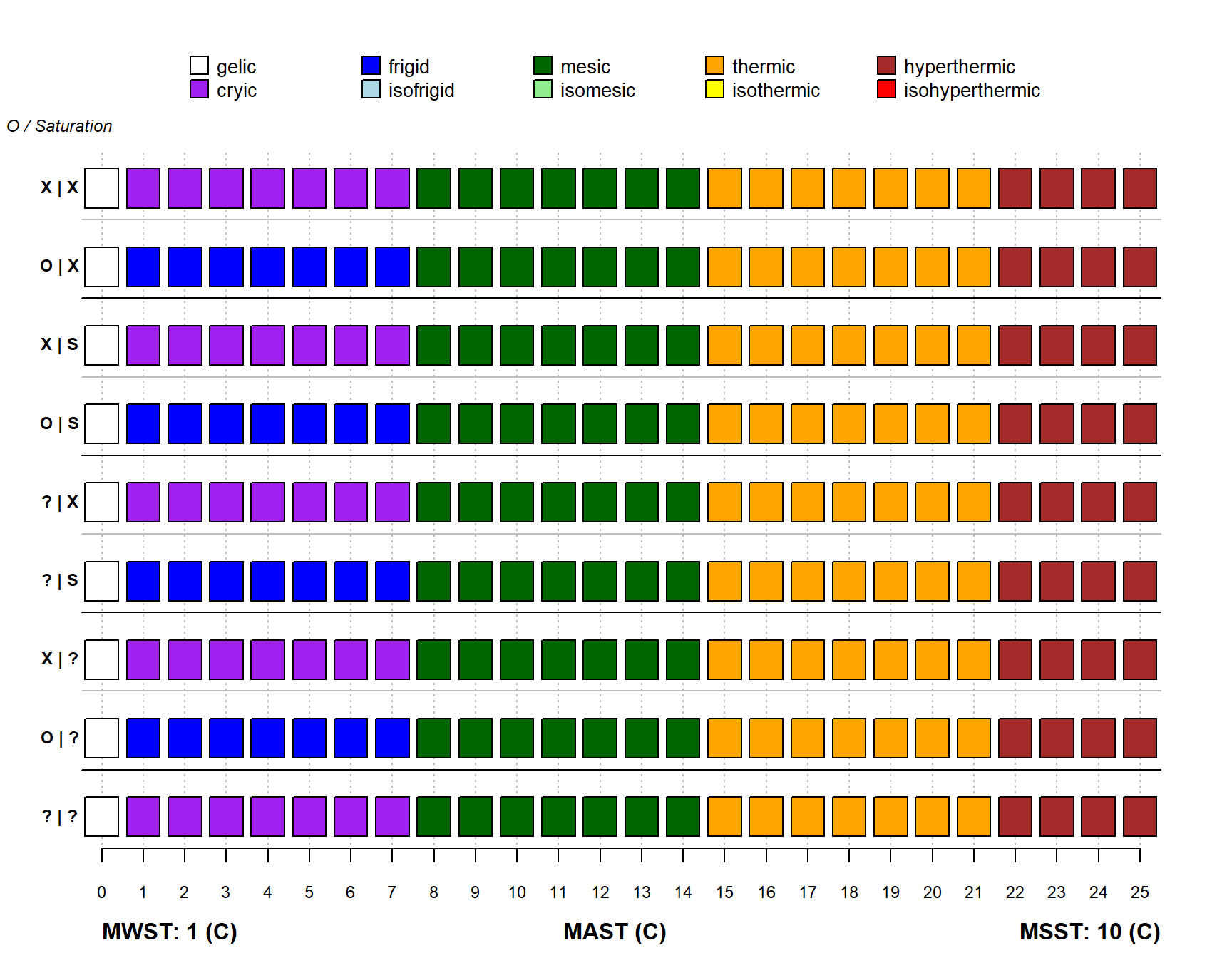``STRplot(mast = 0:25, msst = 9, mwst = 1)``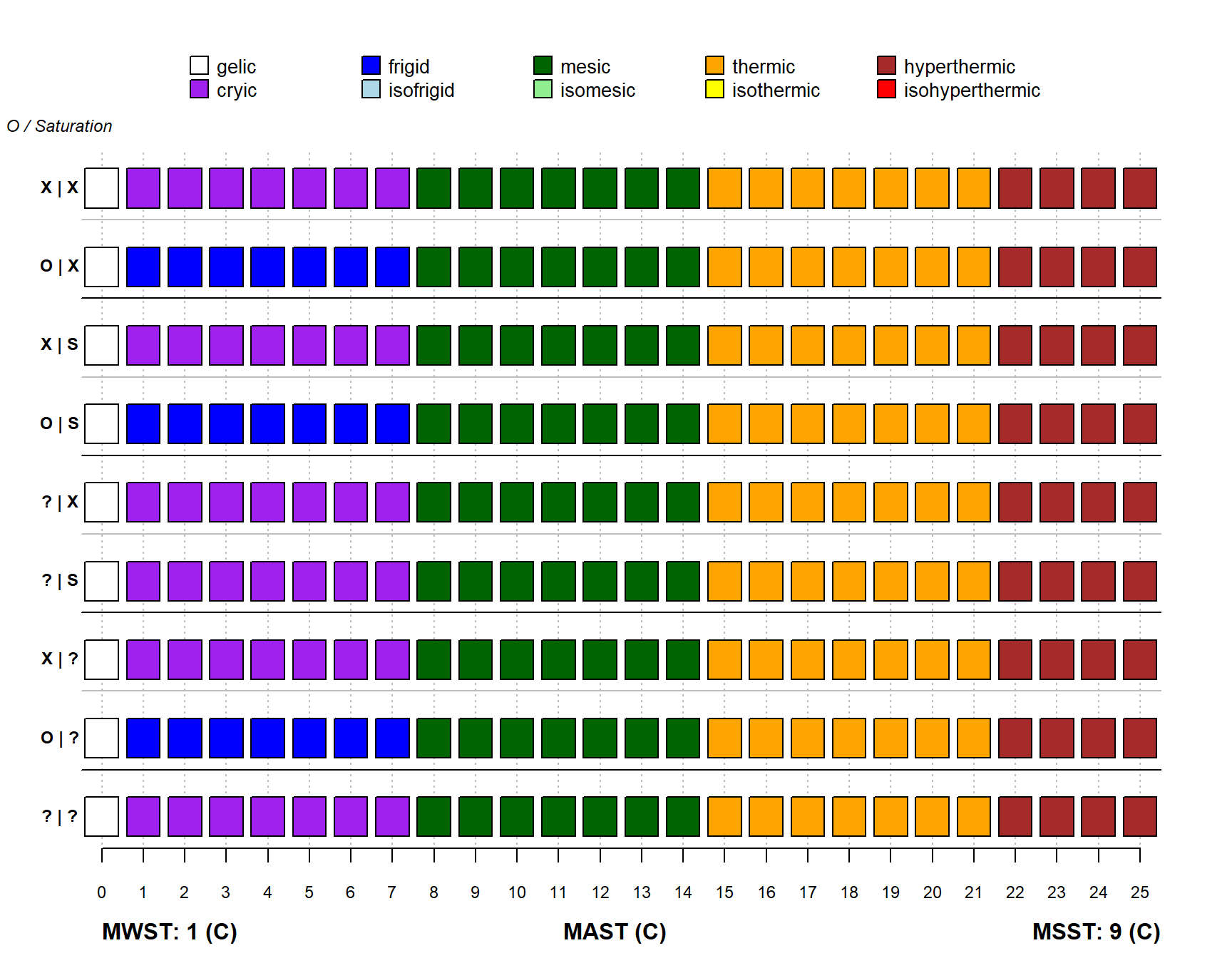``STRplot(mast = 0:25, msst = 7, mwst = 1)``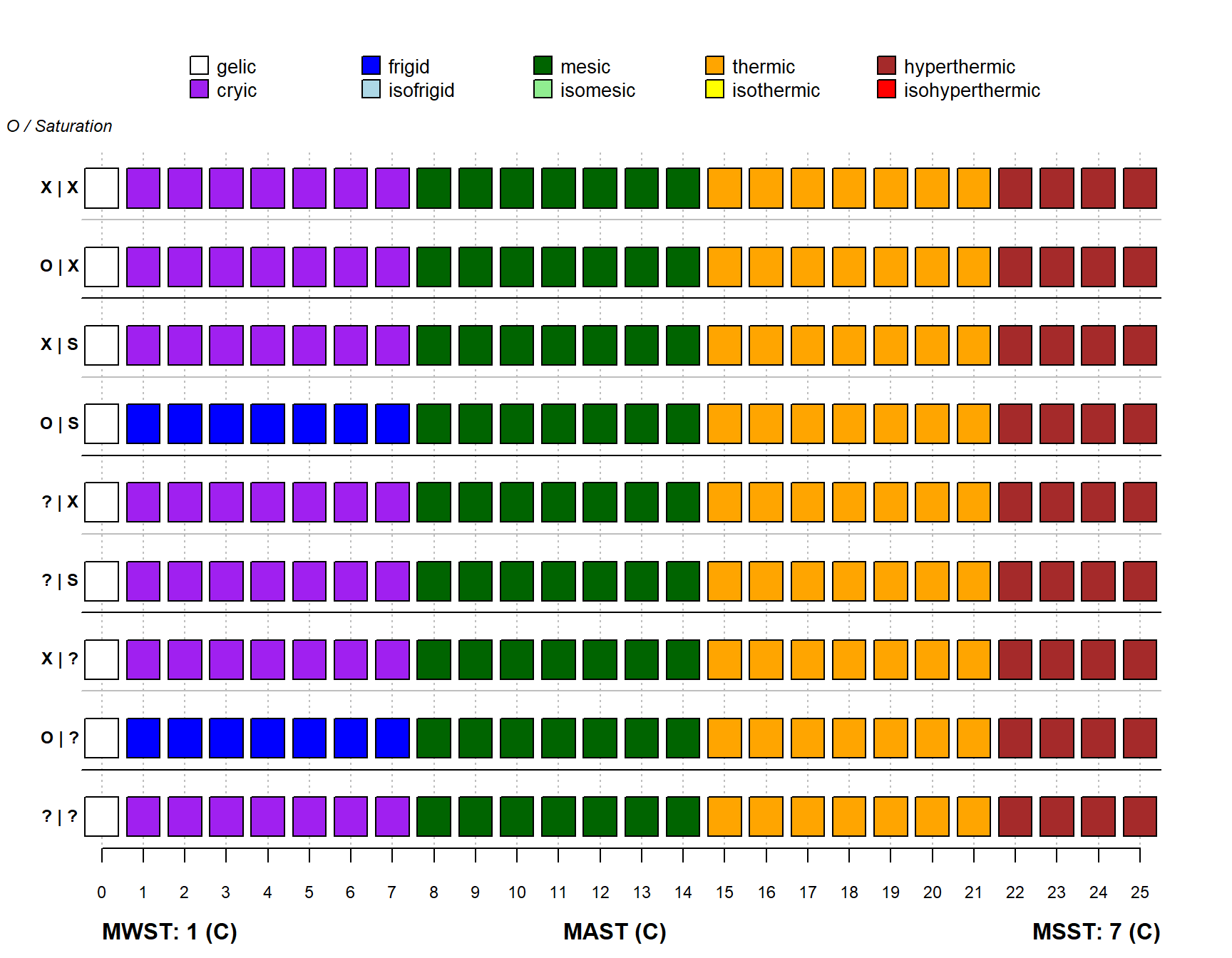``STRplot(mast = 0:25, msst = 6, mwst = 1)``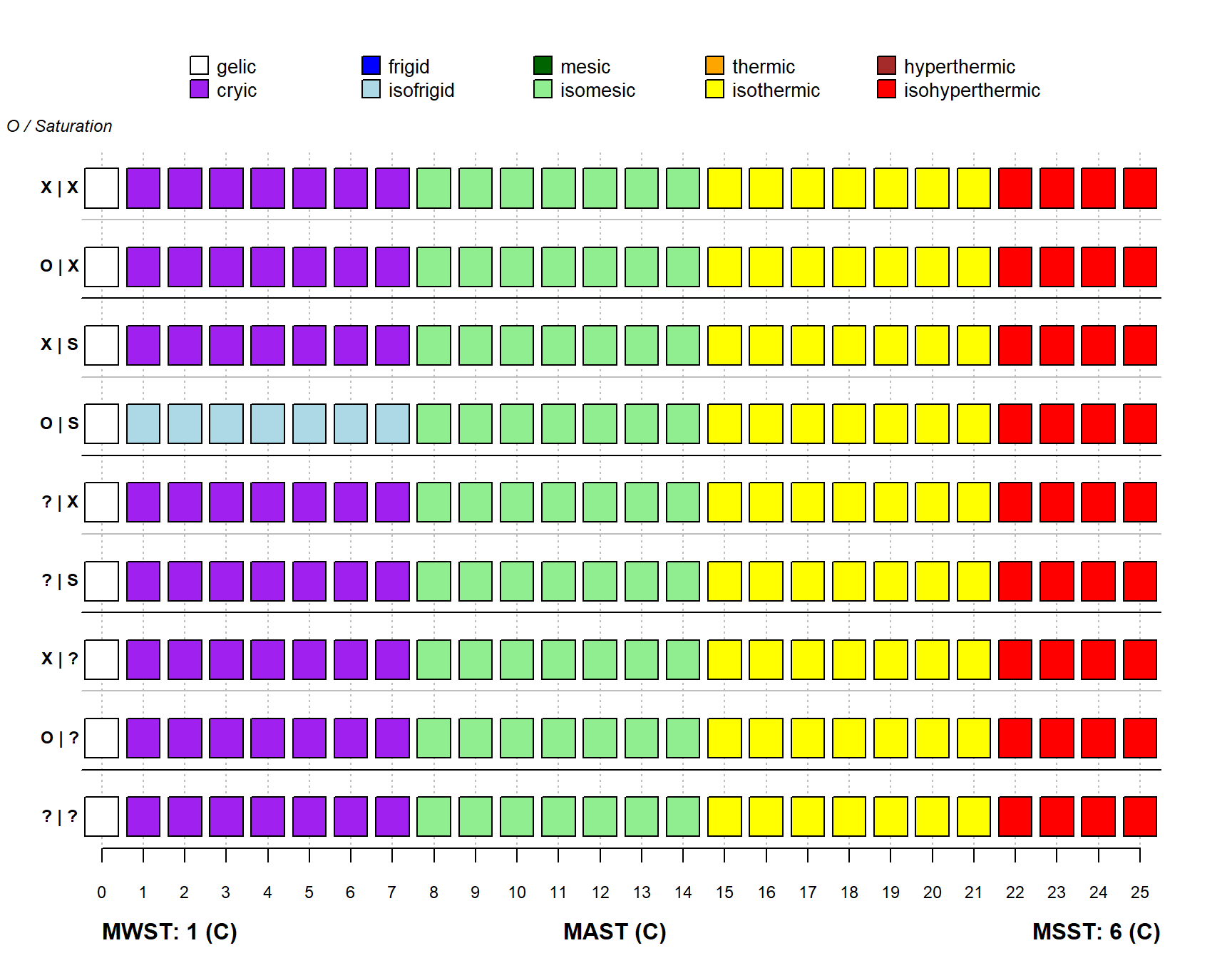``STRplot(mast = 0:25, msst = 5, mwst = 1)``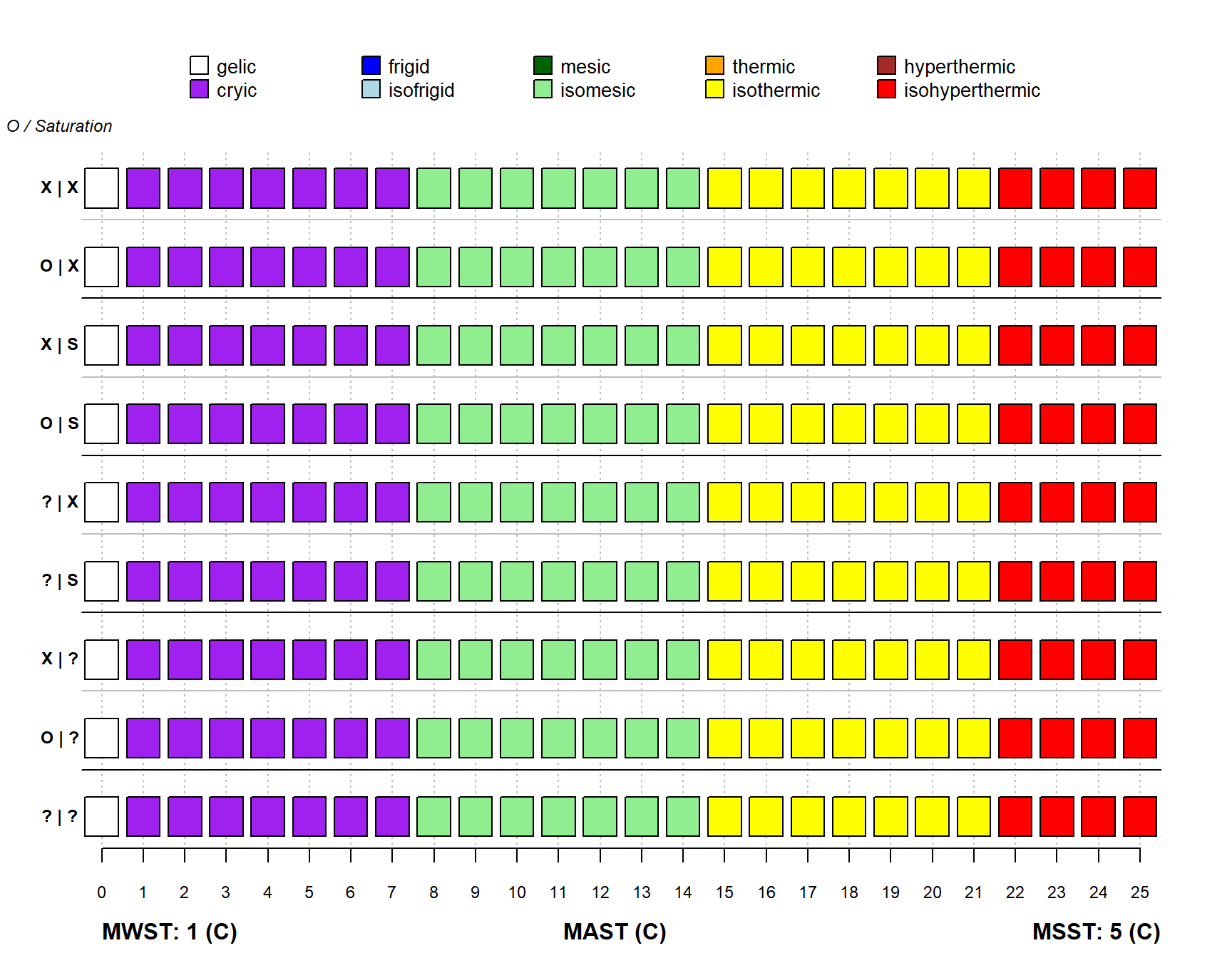``````par(mar=c(4,1,0,1))
STRplot(mast = 5, msst = 1:18, mwst = 0)``````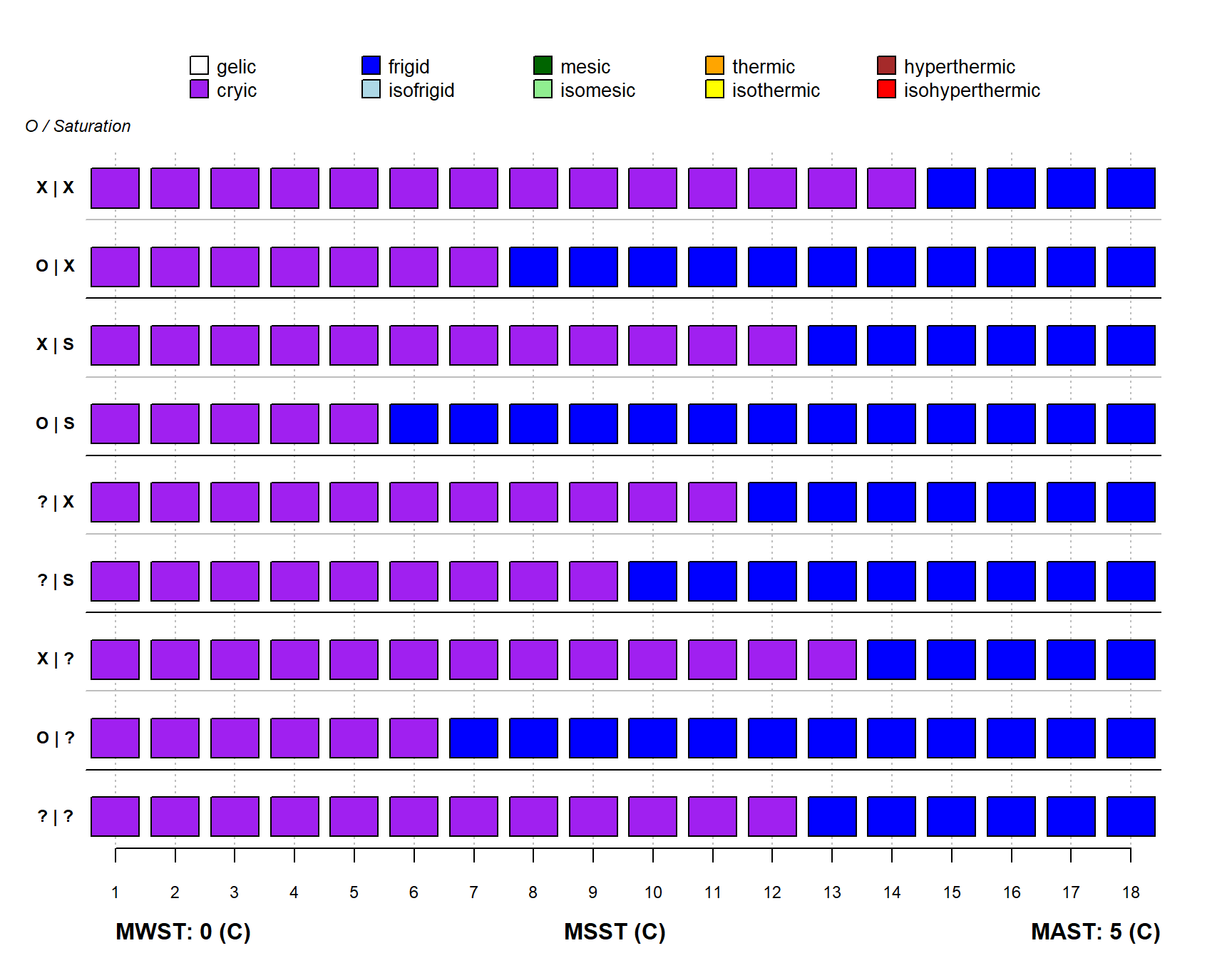``STRplot(mast = 5, msst = 1:18, mwst = 1)``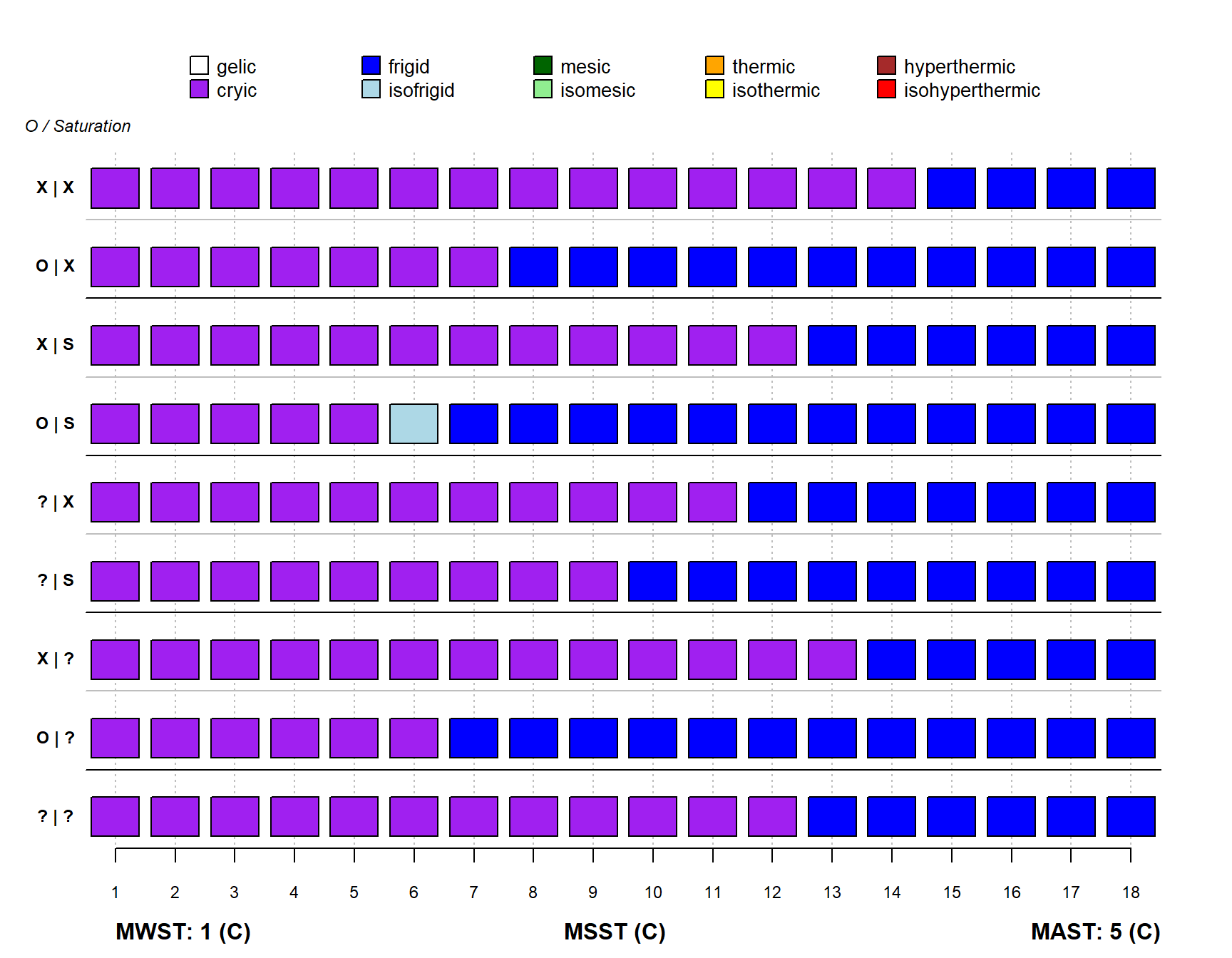``STRplot(mast = 5, msst = 1:18, mwst = 2)``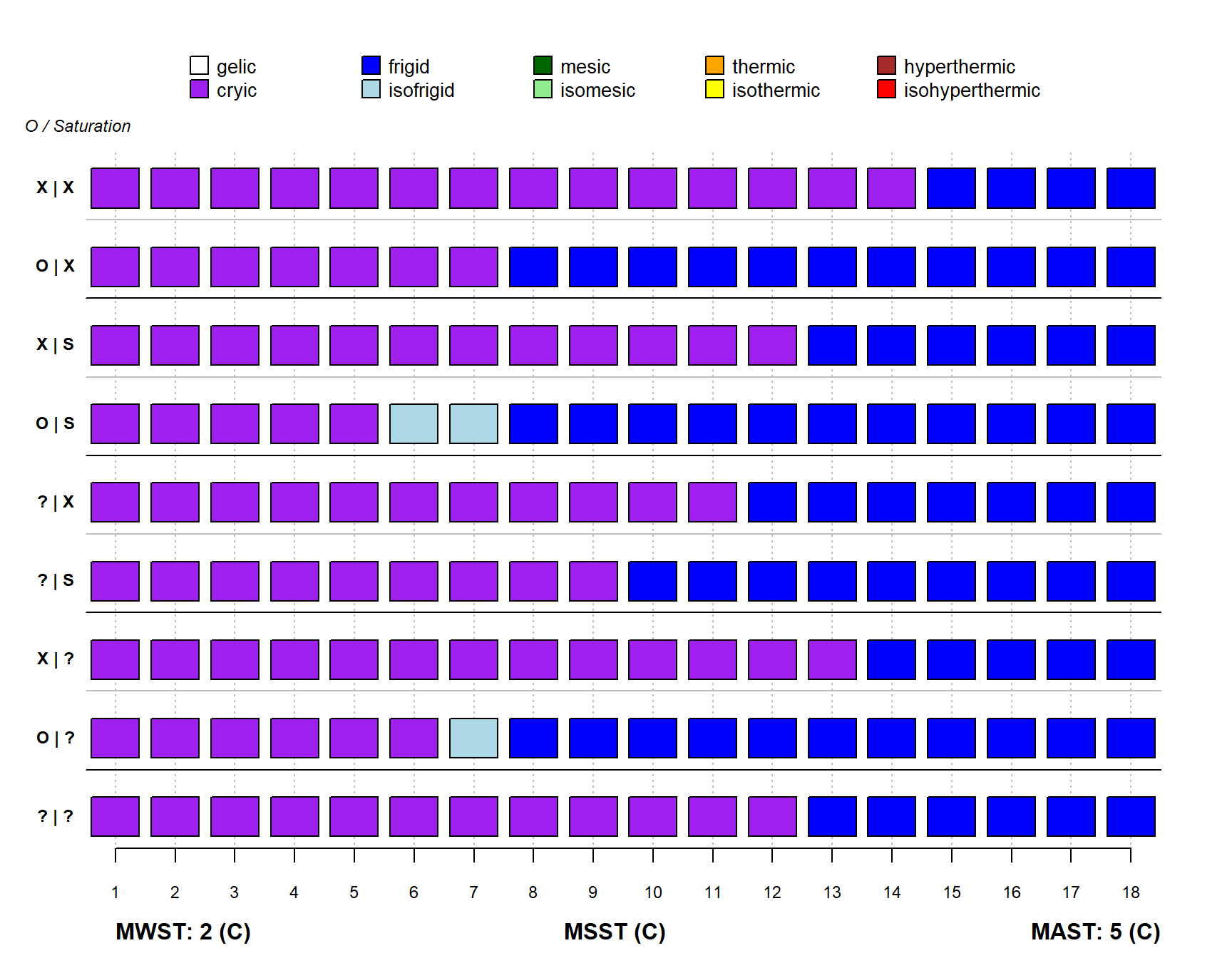``STRplot(mast = 5, msst = 1:18, mwst = 3)``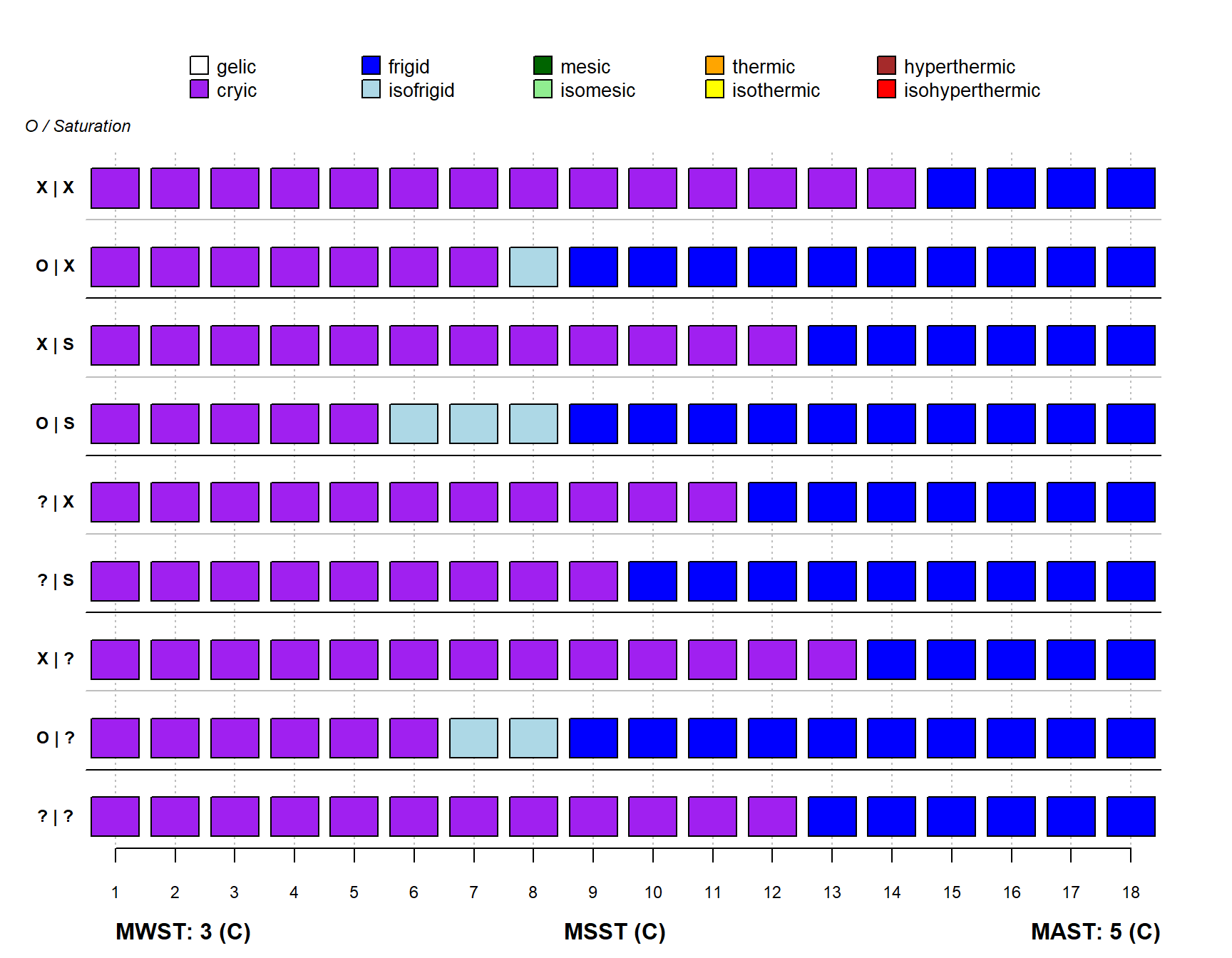``STRplot(mast = 5, msst = 1:18, mwst = 5)``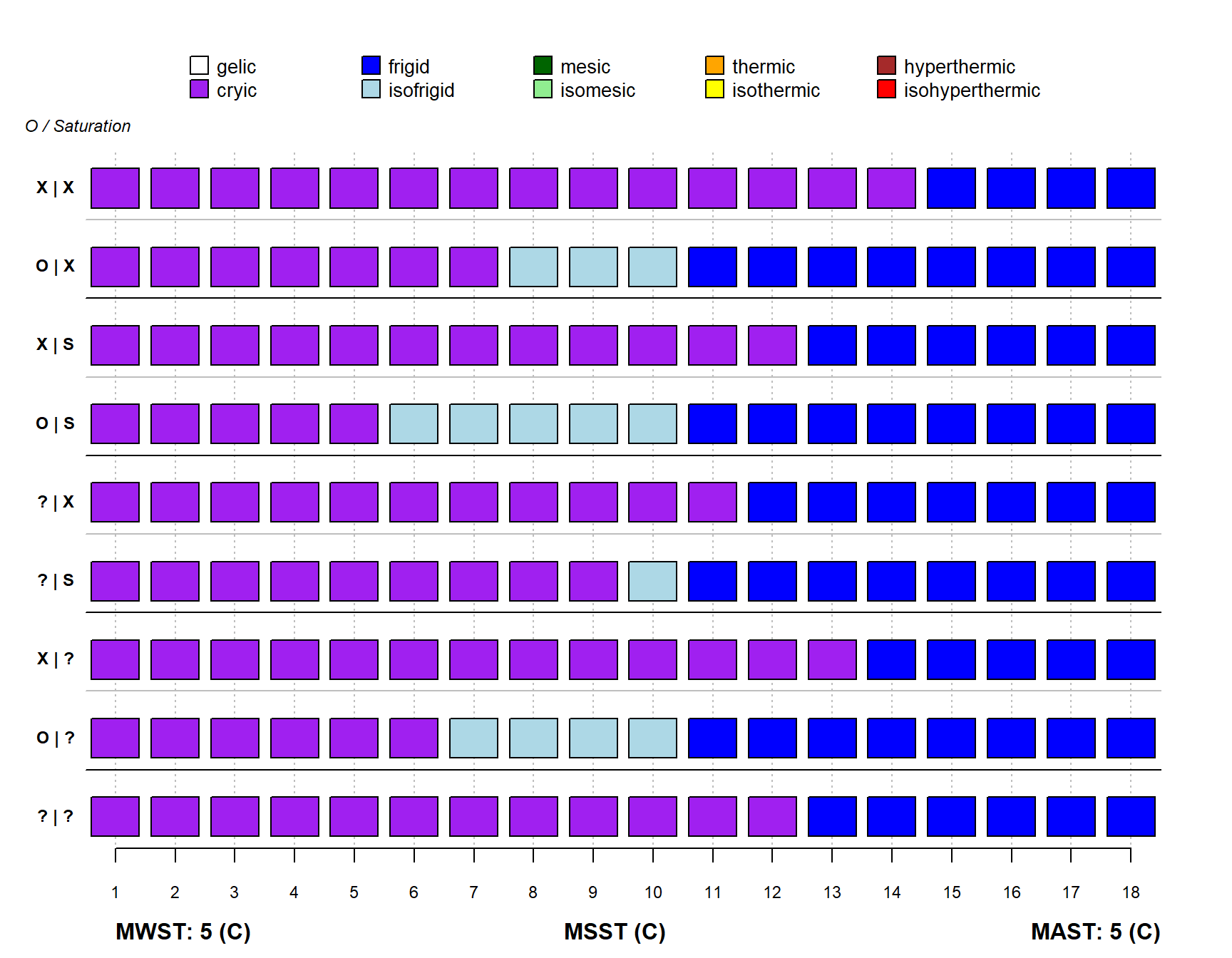``````par(mar=c(4,1,0,1))
STRplot(mast = 10, msst = 1:18, mwst = 5)``````